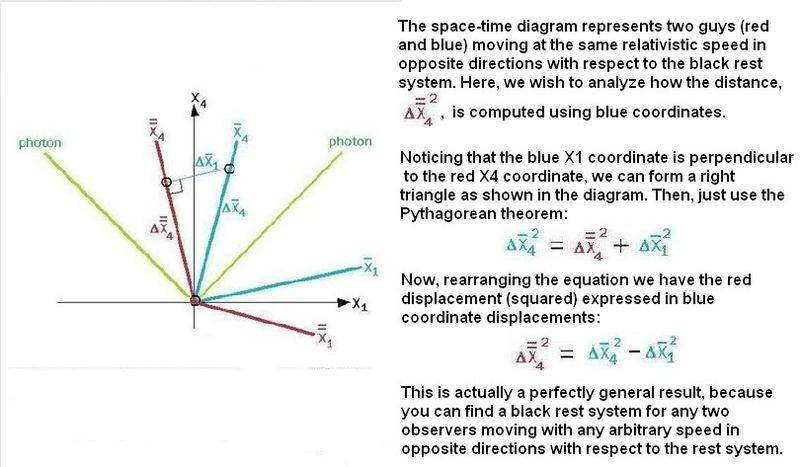# Special Relativity Spacetime Intervals

Just a quick question on spacetime intervals in spacetime. Why is the spacetime interval between two events given by Δs^2 = -c^2(Δt^2) + Δx^2 + Δy^2 + Δz^2, rather than c^2(Δt^2) + Δx^2 + Δy^2 + Δz^2 (as if it were the distance between two points in four spatial dimensions)? Or more succinctly, why is the time component of the spacetime interval negative?

Because the first one is frame invariant (it takes the same value in every frame), whereas the second one is not.

Take the Lorentz Transformation:

$\Delta x'=\gamma (\Delta x-v\Delta t)$
$\Delta t'=\gamma (\Delta t-v\Delta x/c^2)$

Now try calculating $(\Delta x')^2-(c\Delta t')^2$. You'll find that $(\Delta x')^2-(c\Delta t')^2=(\Delta x)^2-(c\Delta t)^2$.

If you try doing the same thing with $(\Delta x')^2+(c\Delta t')^2$ you'll see that $(\Delta x')^2+(c\Delta t')^2 \neq (\Delta x)^2+(c\Delta t)^2$

ghwellsjr
Gold Member
The whole point of the spacetime interval is to arrive at something analogous to a distance in space and/or a period of time that comes out the same no matter what frame you do the calculation in. If you use the second formula, it doesn't come out the same in all frames whereas the first one does.

Just a quick question on spacetime intervals in spacetime. Why is the spacetime interval between two events given by Δs^2 = -c^2(Δt^2) + Δx^2 + Δy^2 + Δz^2, rather than c^2(Δt^2) + Δx^2 + Δy^2 + Δz^2 (as if it were the distance between two points in four spatial dimensions)? Or more succinctly, why is the time component of the spacetime interval negative?

elfmotat and ghwellsjr summed it up pretty well. Here's basically the same point they've made using some graphics.From this point of view you can see why the Pythagorean theorem doesn't work for the blue guy when he tries to compute an invariant value for a red interval.

In a way, the root cause of this situation (from the standpoint of the space-time diagram) has to do with the unique orientation of the blue and red X1 and X4 coordinates. For example, the direction the blue guy moves in the 4-dimensional universe determines the slope of his world line (his X4 coordinate slope with respect to the black rest system).

Now, the mysterious aspect of special relativity is that blue's X1 coordinate (his 3-D cross-section view of the 4-D universe) rotates such that the 45-degree photon world line always bisects the angle between X4 and X1. This is a perfectly general phenomenon, regardless of the observer's speed, holding for any observer moving relative to the black rest system. Accordingly, this explains why the speed of light is always c, for any frame of reference.

So, in a way, you could ask of nature: Why is it that the observer's X1 axis always rotates in that special manner when the X4 rotates? One possible answer that has been suggested is that consciousness can only pick out a cross-section view of the 4-D universe that is intelligible. And The 4-dimensional objects are arranged geometrically so as to present symmetric relationships among objects that are consistent with the laws of physics. Some would argue that this underlies the discovery of laws of physics. And this is consistent with the fact that the laws of physics are the same in all Lorentz frames.

Last edited: